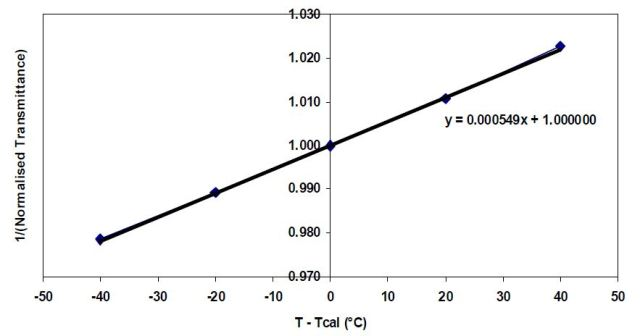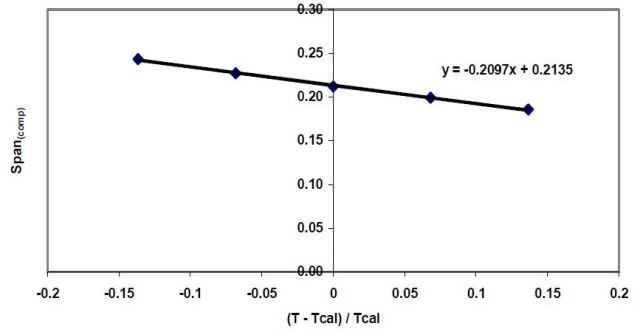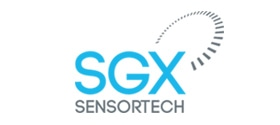# Methods to Determine the Linearization and Temperature Compensation Coefficients

This article covers the methods to determine the temperature compensation and linearization coefficients while calculating target gas concentration with the help of infrared gas sensors. Small changes can occur in these coefficients due to differences in circuit designs. Hence there is a need for new coefficients corresponding to the circuit design of the sensor. A linearized output is obtained over temperature using four coefficients given in the table below.

Table 1. Linearization and Temperature Coefficients

Coefficient Purpose
a Linearisation
n Linearisation
α “Zero” variations over temperature
β “Span” variations over temperature

The “a” and “n” coefficients can be calculated using an appropriate curve fitting program.

## How to Determine “a” and “n” Coefficients

The “a” and “n” coefficients can be determined using the below equation which is derived by altering the equation for calculating the span.

1 – (Act / [Zero x Ref]) = Span x [1 – exp(–aC”)]

where

n = fixed linearization coefficient (to be derived using the curve fitting program)

C = the concentration of the applied calibration test gas in % volume (i.e. 5 for 5% vol.)

a = fixed linearization coefficient (to be derived using the curve fitting program)

Span = the value calculated as part of the calibration process

Ref = the peak-to-peak output of the Reference Detector in volts in the calibration test gas

Act = the peak-to-peak output of the Active Detector in volts in the calibration test gas

Zero = the “Zero” value (Act/Ref in 0% vol. Test gas).

The coefficients can be determined by taking an average of Normalized Absorbance (1 - [Act / (Zero x Ref)]) at ten different concentrations using a minimum of five sensors. The following table shows the data obtained from the IR12BD.

Table 2. Typical Normalized Absorbance Measurements for the IR12BD

Applied Concentration (% vol.) Average Normalised Absorbance
0.0 0.000
0.5 0.031
1.0 0.050
1.5 0.064
2.0 0.076
2.5 0.086
3.0 0.095
3.5 0.103
4.0 0.110
4.5 0.117
5.0 0.123

Upon obtaining the normalized absorbance data, the “a” and “n” coefficients can be determined by means of a curve fitting program using the following equation:

y = s*(1 - exp[-a*(x^n)])

where

y = Average Normalized Absorbance at each concentration.

x = Methane Concentrations

## How to Calculate Temperature Coefficients

Two levels of compensation – alpha compensation and beta compensation – are required to validate changes in temperature. Alpha compensation is used for evaluating the apparent changes in the Zero reading, while the beta compensation is used for evaluating the apparent changes in the Span reading.

Alpha and beta values are determined to convert the following equations in the form of “y = mx + c” so as to obtain a temperature compensated concentration.

Alpha Compensation (Equation 1)

Normalised Transmittance (comp) = Normalised Transmittance x (1 + α (T - Tcal)

where,

Normalized Transmittance = Act / (Zero x Ref), or

Normalized Transmittance = 1 – Normalized Absorbance

Act = the peak-to-peak output of the Active Detector in volts (in zero gas).

Zero = the “Zero” value calculated during the calibration routine.

Ref = the peak-to-peak output of the Reference Detector in volts (in zero gas).

α = the Alpha coefficient.

T = the actual temperature measured at the sensor in kelvin.

Tcal = the temperature (stored in non-volatile memory) measured during the calibration routine (see below) in kelvin.

Beta Compensation (Equation 2)

Span(comp) = Span + [ β x ((T – Tcal) / Tcal)]

where

Span = the “Span” value calculated during the calibration routine.

β = the Beta coefficient.

T = the actual temperature measured at the sensor in kelvin.

Tcal = the temperature measured during the calibration routine in kelvin.

## How to Calculate Alpha Temperature Coefficient

Equation 1 can be rearranged to change the Alpha coefficient equation to a “y = m x + c” form as follows.

(Normalised Transmittance (comp) / Normalised Transmittance) = α (T - Tcal) + 1

The target value of the normalized transmittance calculation is equated to 1 i.e. 0% volume concentration as the alpha coefficient temperature compensates the zero reading.

(1 / Normalised Transmittance) = α (T - Tcal) + 1

where

y = (1 / Normalised Transmittance)

m = α

x = (T - Tcal)

c = 1

The values of x and y are needed to calculate the alpha coefficient. The x and y are determined by taking the average of Normalized Transmittance for at least two different ambient temperatures on a minimum of five sensors. The table below shows the data obtained from the IR12BD.

Table 3. Typical Normalized Transmittance Measurements for the IR12BD at Various Temperatures

Temperature (°C) Average Normalised Transmittance (to 0% vol. Target Gas)
60 0.978
40 0.989
20 1.000
0 1.011
-20 1.022

Subsequently, a graph of "T - Tcal" along x-axis and "1/(Normalized Transmittance)" along y-axis should be plotted to determine alpha.Figure 1. Graph to Determine Alpha

The value of alpha from the graph is 0.000549.

## How to Determine Beta Temperature Coefficient

Equation 2 can be rearranged to change the beta coefficient equation into a “y = mx + c” form as follows:

Span(comp) = β x [(T – Tcal) / Tcal] + Span

where

y = Span(comp)

m = β

x = [(T – Tcal) / Tcal]

c = Span

Note: Span = (1 – Normalized Transmittance) / (1 – exp(-aCn))

In order to calculate the beta coefficient, the values of x and y need to determined. The x and y values can be calculated taking an average of Normalized Transmittance for at least two different ambient temperatures on five sensors. The data obtained from the IR12BD is given in the table below.

Table 4. Typical Normalized Transmittance Measurements for the IR12BD at Various Temperatures

Temperature (°C) Average Normalised Transmittance (to 5% vol. Methane) Span(comp)
60 0.873 0.186
40 0.877 0.199
20 0.880 0.212
0 0.883 0.227
-20 0.885 0.243

The beta value can be calculated from the graph having "(T - Tcal) / Tcal” on x-axis and "Span(comp)" along y-axis.Figure 2. Graph to Determine Beta

The value of beta from the graph is –0.2097.

SGX Sensortech is a market leader in innovative sensor and detector devices that offer unrivalled performance, robustness and cost- effectiveness.

SGX have been designing and manufacturing gas sensors for use in industrial applications for over 50 years, offering excellent applications support for an extensive range of gas sensors and the expert capability for custom design or own label.

As an independent OEM supplier of gas sensors, we pride ourselves on providing customers with unrivalled product reliability and personal product support via specialist engineers.

SGX gas sensors are built to the highest standards with all pellistor and infrared gas sensors achieving ATEX and IECEx certification, SGX gas sensors are also UL and CSA approved.

Our product portfolio has continued to expand in technology and detectable gases used in a wide range of applications including:-

• Mining
• Oil and gas
• Confined space entry
• Indoor air quality
• Industrial area protection
• Leak detectionThis information has been sourced, reviewed and adapted from materials provided by SGX Sensortech (IS) Ltd.

## Citations

• APA

SGX EUROPE SP. Z O.O.. (2020, March 13). Methods to Determine the Linearization and Temperature Compensation Coefficients. AZoSensors. Retrieved on August 04, 2020 from https://www.azosensors.com/article.aspx?ArticleID=541.

• MLA

SGX EUROPE SP. Z O.O.. "Methods to Determine the Linearization and Temperature Compensation Coefficients". AZoSensors. 04 August 2020. <https://www.azosensors.com/article.aspx?ArticleID=541>.

• Chicago

SGX EUROPE SP. Z O.O.. "Methods to Determine the Linearization and Temperature Compensation Coefficients". AZoSensors. https://www.azosensors.com/article.aspx?ArticleID=541. (accessed August 04, 2020).

• Harvard

SGX EUROPE SP. Z O.O.. 2020. Methods to Determine the Linearization and Temperature Compensation Coefficients. AZoSensors, viewed 04 August 2020, https://www.azosensors.com/article.aspx?ArticleID=541.